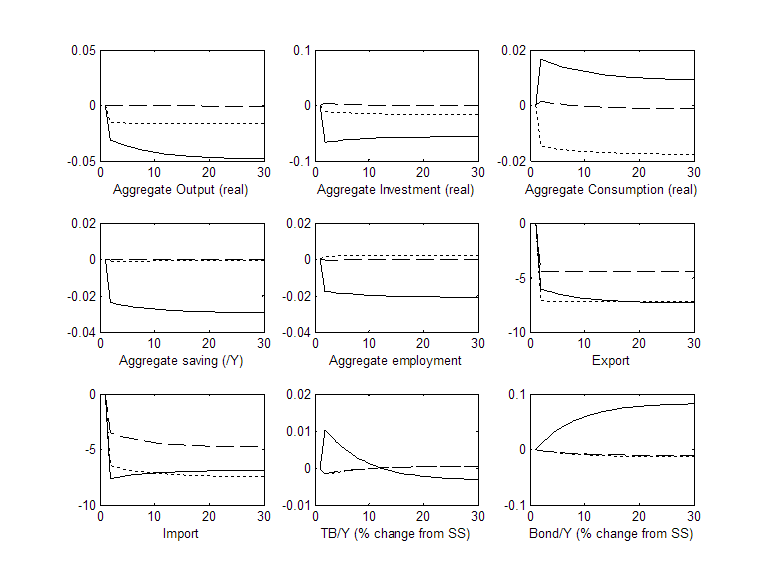Sunghyun Henry Kim
Serge Shikher
ECONOMICS WORKING PAPER SERIES
Working Paper 2017–10–B
500 E Street SW
Washington, DC 20436
October 2017

Office of Economics working papers are the result of ongoing professional research of USITC Staff and are solely meant to represent the opinions and professional research of individual authors. These papers are not meant to represent in any way the views of the U.S. International Trade Commission or any of its individual Commissioners. Working papers are circulated to promote the active exchange of ideas between USITC Staff and recognized experts outside the USITC and to promote professional development of Office Staff by encouraging outside professional critique of staff research.

Sunghyun Henry Kim, Serge Shikher
Office of Economics Working Paper 2017-10-B
October 2017

Abstract

Sunghyun Henry Kim
Department of Economics
Sungkyunkwan University
shenrykim@skku.edu

Serge Shikher
Research Division
Office of Economics
serge.shikher@usitc.gov

### 1 Introduction

There is a newfound interest in the macroeconomic effects of trade policies. There has been much discussion in the media regarding US trade deficits and use of tariffs and border adjustment tax (BAT) to affect trade balance. If persistent US trade deficits are viewed with concern, can tariffs or other protectionist policies be considered as tools for improving the trade balance?1

In order to answer this question, a model that combines realistic modeling of macroeconomic phenomena and international trade is needed. Traditionally, macro models focus on intertemporal allocation of resources while trade models focus on inter-sectoral allocation of resources and changes in trade patterns. In this paper, we try to reconcile these two modeling worlds.

Toward this goal, we construct a dynamic general equilibrium model for trade analysis. The model is based on optimizing behavior of agents that are tied together in a general equilibrium framework. Importantly, households in the model optimize across time, so that savings, investment, and labor supply decisions are endogenous. By contrast, a typical computable general equilibrium (CGE) model of trade includes only static optimization. Development of computational software and solution methods makes it possible to adopt complicated dynamic trade models to explore intertemporal nature of macroeconomic responses to trade shocks as opposed to typical CGE models which lack these features.2

The model provides a laboratory environment in which we can conduct computational experiments to evaluate macroeconomic effects and welfare implications of various trade policies. The model is a large-scale three country model with two sectors.3 It features capital accumulation with adjustment costs and international bond trading. Trading in commodities and assets allow the model to endogenously capture both optimal intertemporal and intratemporal allocation of resources. The three country setup allows us to endogenously calculate trade diversion and creation effects when trade policies change. A two country model cannot provide the analysis on how bilateral trade policies would affect trade with the third country. The model incorporates trade costs in a manner similar to the gravity model. Trade costs include both policy-related and non-policy related costs.

A key feature of the model is its realistic representation of international financial markets. Most DGE models feature complete asset markets, so that countries can insure against any eventuality. This results in zero or very small changes in current account in response to trade policy changes. While the assumption of complete asset markets significantly simplifies model solution, it is not suitable for modeling of trade balance (Obstfeld2012). On the other hand, modeling incomplete asset markets presents significant computational challenges, which we were able to overcome in this paper. In order to solve the model, we employ a linear approximation method based on a shooting algorithm similar to the one used in Mendoza and Tesar (1998), Gorodnichenko, Mendoza and Tesar (2012), and Kim and Kose (2014).4 This method allows us to analyze how one-time permanent changes in tariff rates affect macroeconomic variables in the model over time.

The three countries in the model economy are calibrated to US, China and the ROW.5 More generally, the model can be used to analyze many potentially important trade issues when calibrated to different countries or regions. We use the model to study the effects of an increase in US tariff to 10% on (a) Chinese goods and (b) goods from all countries. Modeling results from (a) show that US imports from China fall and US imports from the ROW increase. US exports fall because of an increase in US producer prices. US trade balance improves in the short run, but returns to the initial state (or a level slightly below it) after about 13 years. Due to the temporary improvement in US trade balance, US increases its foreign asset position. Both savings and investment fall in the US, but the initial fall in investment is greater than the fall in savings. US wage in the tradable goods sector increases temporarily but then returns to its original level. The effect on welfare is negative. The positive temporary effect of higher tariffs on US trade balance is much greater when tariffs are imposed on all imports than just imports from China.

This paper contributes to the small, but growing literature investigating the relationships between trade and macroeconomic phenomena using dynamic simulation models. Lindé and Pescatori (2017) use a two-country one-sector new-Keynesian DSGE model to study the robustness of the Lerner symmetry result to various model assumptions. Erceg, Prestipino and Raffo (2017) also use a two-country one-sector new-Keynesian DSGE model to study the effects of import tariff, export subsidy, and border adjustment tax (BAT) on output, exchange rate, and inflation. Eaton, Kortum, Neiman and Romalis (2016) use a multi-country general equilibrium model with capital to explain how much certain shocks have contributed to a decline in worldwide trade following the Great Recession. Their model, however, has complete markets and fixed labor. Kim and Kose (2014) uses a multi-sector small open economy general equilibrium model with capital, labor and incomplete markets with bonds. They use a shooting algorithm to analyze how permanent changes in tariff rates affect fiscal budget and welfare. The model in our paper extends the model of Kim and Kose (2014) to include three countries and international trade costs.6

### 2 Model

There are three countries $n = 1, 2, 3$ and two sectors $j = 1, 2$ in each country. Sector 1 goods are traded while sector 2 goods are not traded. We have in mind that country 1 is the US, 2 is China, and 3 is ROW. In order to allow two-way trade between countries, we use the Armington assumption that goods are differentiated by country of production. Therefore, sector 1 goods from country 1 are not perfectly substitutable with sector 1 goods from country 2. Shipping goods between countries involves paying trade costs. Some of these costs are policy-related, for example tariffs. The economies of all three countries have the same structure.

The model includes households, firms, and governments. Households possess capital and receive labor and rental income from the firm. We assume that capital goods used for production of goods in both sectors are domestically produced. Each sector uses two factors of production, capital and labor, to produce output. Capital is specific to each sector but labor is mobile between the two sectors. In equilibrium, nominal wage and rental returns in the two sectors are the same within a country.

The government in each country must finance an exogenous stream of expenditures through revenues from domestic lump sum taxes and tariffs on imported goods. Households are able to borrow and lend on international markets using one-period risk-free bonds, so the current and financial account balances are endogenous.

#### 2.1 Households

Households in each country $n$ consume four goods: sector 1 goods from the three source countries and domestic sector 2 good. We reduce the four-good optimization problem into a two good problem by defining the composite tradable consumption good $Cn1t$ and nontradable good $cn2t$. A representative household in each country $n$ chooses its consumption of these two goods and its labor input to maximize a constantrelative risk aversion (CRRA) utility function:

 (1)

subject to the budget constraint

 $Y nt + pn2tTnt + Pn1tBn,t-1 = Pn1tCn1t + pn2tcn2t + Int + RtPn1tBnt,$ (2)

where $βt$ is the discount factor, $hnjt$ is the labor input, $σ$, $φ$, and $ξ$ are parameters, $Y nt$ is the total factor income (defined in equation (3) below), $pn2t$ is the price of sector $2$ (nontradable) good produced in $n$, $Pn1t$ is the price of sector 1 composite tradable consumption good ($Cn1t)$, $Tnt$ denotes net transfers from government in a lump-sum fashion (assumed to be in the nontradable good for simplicity), $Bnt$ denotes the quantity of discounted bonds purchased in period $t$ maturing in $t + 1$ with price $Rt$, and $Int$ is the total value of investment (defined in equation (4) below). Note that the composite consumption good and its price are denoted with upper case letter. Bonds are priced in terms of sector 1 composite good price $Pn1t.$ Under financial autarky, $Bnt$ is set to zero in every period.

Total factor income $Y nt$ of households consists of labor and capital incomes in each sector:

 $Y nt = ∑ jpnjt(wnjthnjt + rnjtknjt),$ (3)

where $wnjt$, $rnjt$, $hnjt$, $knjt$ are the (real) wage, (real) rental rate, labor input, and capital. Note that price is denoted by lower case $p$ in order to emphasize that this price is not the price of a composite consumption good but the price of sector $j$ good produced in country $n$. Investment spending goes toward buying investment (capital) goods in each sector:

 $Int = ∑ jpnjtinjt,$ (4)

where $injt$ is the (real) investment in sector $j$.

Households in country $n$ accumulate capital in each sector $j$ according to the following equation:

 $knj,t+1 = (1 - δj)knjt + knjtϕ injt knjt ,$ (5)

where $δj$ is the depreciation rate and $ϕ$is adjustment cost function ($ϕ > 0$, $ϕ′ > 0$, $ϕ′′ < 0)$ as in Baxter and Crucini (1993).

Consumers have CES preferences over tradable consumption goods from all countries of origin $i$:

 (6)

where $cin1$ is the consumption in country $n$ of tradable sector $1$ good produced in $i$, and $bin$ is the preference parameter. Another way to interpret the consumption structure is that households consume the composite consumption good $Cn1t$ which is produced by three intermediate inputs from all countries $cin1t$. In this case, equation (6) is interpreted as a production function of the final consumption good rather than a preference function.

Shipping goods between countries incurs trade costs. In order to deliver $1 of good $j$ to country $n$, country $i$ must ship $dinj ≥ 1$ dollars of this good. Therefore, trade cost is ad-valorem or “iceberg”.7 We measure international trade costs relative to domestic trade costs, so $dnnj = 1$. The price of good $j$ produced in country $i$ is $pij$ when it leaves the factory. Therefore, $pij$ is the producer price or factory-gate price. When this product arrives to country $n$, its price is $pinjt = pijtdinjt$. Price $pinjt$ is the consumer or delivered price of the good. Trade cost $d$ includes all costs of delivering goods from one country to another, such as freight, insurance, security, tariffs, and non-tariff barriers. Trade costs are specific to each country pair, direction of trade, and industry. Trade costs are estimated from observed trade flows, as in the gravity literature. We derive the gravity equation in Section 2.5. Tariffs are part of trade costs. We denote by $τinjt$ the tariffs imposed by country $n$ on industry $j$ good produced in country $i$. We have  $dinjt = τinjt + dinjt¯,$ where $dinjt¯ ≥ 1$ are all trade costs other than tariffs. We define  $Xinjt = cinjtpinjt = cinjtpijtdinjt,$ (7) as the total spending by country $n$ on industry $j$ good produced in country $i$. Focusing on the tradable sector, we define $Xn1t$ as the spending on tradable composite consumption good in country $n$:  $Xn1t = Pn1tCn1t = ∑ iXin1t = ∑ icin1tpi1tdin1t.$ (8) Maximizing (6) subject to (8) yields the equilibrium expressions of relative demand for each consumption good in sector 1 and the price of the tradable composite consumption good: $Xin1t = bin1 γ pi1tdin1t Pn1t γ-1 γ Xn1t, (9) Pn1t = ∑ ibin1 γ pi1tdin1t γ-1 γ γ γ-1 . (10)$ #### 2.2 Firms The production function in each country and sector is  $ynjt = Anjtknjtαj hnjt1-αj ,$ (11) where $Anjt$ is the level of sector-specific productivity. The productivities are assumed to be constant at one since we consider a deterministic environment. The no-profit conditions imply that  $pnjtynjt = pnjtrnjtknjt + pnjtwnjthnjt$ (12) for every sector $j$, country $n$, and period $t$. #### 2.3 Government Government revenue is from tariff income. The government budget constraint is  $∑ iτin1tpi1tcin1t = pn2tGnt + pn2tTnt,$ (13) where $Gnt$ denotes exogenous government spending and $Tnt$ denotes lump-sum fiscal transfers to households, which can be interpreted as a lump-sum tax if negative. Note that we assume that government spending is in nontraded goods only for simplicity. Also note that there are no tariffs collected in sector 2 since its good is non-traded. #### 2.4 Market clearing conditions We impose market clearing conditions on all goods in the model. For nontradable sector 2, the market clearing condition for each country $n$ is  $yn2t = cn2t + in2t + Gnt.$ (14) For tradable sector 1, each country $n$ has the following market clearing condition:  $pn1tyn1t + Pn1tBn,t-1 = ∑ idin1t¯pi1tcin1t + pn1tin1t + RtPn1tBnt,$ (15) which can be derived by combining the government budget constraint (13), households budget constraint (2), and market clearing condition for nontraded good (14). For tradable sector 1, worldwide resource constrains for each good $i$ are:  $pi1tyi1t = ∑ ndin1t¯pi1tcin1t + pi1tii1t.$ (16) This condition, which must hold for each sector $i$ in every period $t$, says that the value of output must equal to total spending in that sector. Once all three market clearing conditions hold, the following bond market clearing condition holds for every period $t$ (Walras’s law):  $∑ nPn1t(RtBnt - Bn,t-1) = 0.$ (17) Since the above expression is in fact equal to trade balance, this condition says that trade balance of the three countries should sum up to zero. When we solve the model, we set the price of sector 1 good in country 1, $p11$, equal to 1, which means that this good is the numeraire. #### 2.5 Gravity We now derive the gravity equations in our model. Using the demand equation (9) we rewrite the market clearing condition in the tradable sector: $Y i1t = ∑ nXin1t + Ii1t = = ∑ n bin1 γ pi1tdin1t Pn1t γ-1 γ Xn1t + Ii1t = = bin1 γ pi1tγ-1 γ ∑ n din1t Pn1t γ-1 γ Xn1t + Ii1t (18)$ Rearranging, we obtain  $bin1 γ pi1tγ-1 γ = Y i1t - Ii1t ∑ n din1t Pn1t γ-1 γ Xn1t$ (19) Dividing both numerator and denominator by $Y 1t = ∑ iY i1t$, and defining  $Πi1tγ-1 γ ≡∑ n din1t Pn1t γ-1 γ Xn1t Y 1t$ (20) we obtain  $bin1 γ pi1tγ-1 γ = Y i1t - Ii1t ∕Y 1t Πi1tγ-1 γ$ (21) Using (21), the demand equation (9) can be rewritten as $Xin1t = bin1 γ pi1tγ-1 γ din1t Pn1t γ-1 γ Xn1t = Y i1t - Ii1t Xn1t Y 1t din1t Pn1tΠi1t γ-1 γ (22)$ and the price index (10) can be rewritten as  $Pn1tγ-1 γ = ∑ i din1t Πi1t γ-1 γ Y i1t - Ii1t Y 1t$ (23) Equation (22) is the theoretical gravity equation that says that bilateral trade flows $Xin1t$ are proportional to the income of importer $i$ and spending of exporter $n$, and inversely proportional to the trade cost between them. The theoretical gravity equation also includes price indices $Pn1t$ and $Πi1t$. Price index $Pn1t$ was called by Anderson and van Wincoop (2003) the inward multilateral resistance, while price index $Πi1t$ was called the outward multilateral resistance. The gravity equation (22) can be used to estimate trade costs $din1t$ from observed trade flows $Xin1t$, as described in Piermartini and Yotov (2016). Equations (22), (20), and (23) form the structural gravity system. ### 3 Calibration We calibrate the benchmark model to capture the main features of the data for US and China. Some parameters are the same in all three countries, while other parameters are country-specific. #### 3.1 Common parameters We fix the value of $β$ at 0.96 to match the steady state level of the annual world real interest rate at 4 %. The inverse of the Frisch elasticity of the labor supply $ξ$ is set at 2.5, suggesting a low Frisch elasticity that is close to the value used in Smets and Wouters (2007). The labor disutility parameter $φ$ is set to ensure that the steady state value of hours worked (i.e., the sum of hours worked in the tradable and nontradable sectors) is one third. The value of the risk aversion parameter $σ$ is set to 2 to match the intertemporal elasticity of substitution in the US data, which is close to the average value reported in the panel study by Ostry and Reinhart (1992). The value of $γ$ is set at 0.782 to match the elasticity of substitution in the tradable good consumption function at 1.3 which is very close to the value used by Mendoza (1992) and Ostry and Reinhart (1992). Capital share in the export good production $α1$ is set at 0.4 and the capital share in the nontradable good $α2$ is set at 0.3. Depreciation rate is set at 10%. The parameter value for $ϕ$ in capital adjustment costs is set at $10$ to match the volatility of investment in the data. #### 3.2 Country specific parameters Manufacturing and agriculture goods are assumed to be tradable while services are assumed to be nontradable. The share parameters in the CES consumption function $b$ are set to match the sectoral data for tradable goods consumption in US, China and the ROW, calculated from 2013 COMTRADE data. Consumption shares of import goods from China and ROW in US are 6.5% and 21.2%, respectively. For China, consumption shares of import goods from US and ROW are 1.2% and 8.5%, respectively. For ROW, consumption shares of import goods from US and China are 3.7% and 4.3%, respectively. Trade costs are estimated using a gravity regression using 2013 trade and output (IndStat and FaoStat) data. Domestic trade costs are normalized to 1. Trade costs were estimated for about 50 countries and 30 manufacturing and agriculture industries and we calculate (trade) weighted averages. Total trade costs for each pair are as follows: $China_to_US 1.304US_to_China1.610 China_to_ROW1.899 US_to_ROW: 1.655 ROW_to_China1.350 ROW_to_US 1.186$ Since trade costs include not only tariff rates but all the other trade related costs, we need to separate the tariff related trade costs from the total trade costs. We use the MFN tariff rate for US, China and the world average, which are 2.7%, 4.0%, and 4.0%, respectively. The data are from the World Bank (weighted mean, all products) and the data for US and China is from the year 2015 and the world average is from the year 2012. We set the government spending endogenously to make the lump-sum transfers (taxes) at zero at the steady state. In this way, we can minimize the income effects coming from fiscal sector when tariff rates change. The initial asset holding position ($p1B1∕pxyx,$ $p2B2∕pmym)$ for US and China are set to match the steady state trade balance at zero. We can change the steady state trade balance according to the data (-4.04% of GDP for US and 4.41% of GDP for China in 2016), but the results are similar to the case with zero trade balance as we focus on how much trade balance moves from the steady state. #### 3.3 Model solution We analyze how changes in tariff rates affect both steady state and transitional dynamics of the model economy (between the pre- and post-tariff reform states). We assume that changes in tariff rates are permanent implying that we only consider time-invariant one-time changes in tariff rates. In order to solve for the model dynamics, we employ a linear approximation method based on a shooting algorithm. Linear approximation incurs large approximation errors if the model moves away from the deterministic steady state where the approximation is performed around. In order to minimize the approximation errors, we need to approximate the model around the new steady state because changes in tariff rates are permanent. However, the value of asset holdings at the new steady state is not known because of the well-known indeterminacy issue in small open economy models with incomplete financial markets.8 Shooting algorithm uses an iteration method to pin down the new steady state asset holding position that is consistent with the debt-accumulation dynamics of the existing steady state equilibrium. These procedures are well documented in Kim and Kose (2014). Appendix describes the detailed equations for the model solution including steady state and linearized first order conditions. ### 4 Simulation results We experiment how an increase in tariff rates in US against import goods from China and/or ROW affect macroeconomic dynamics, especially trade balance and inter-sectoral resource movement. #### 4.1 Increase in tariff rates against Chinese import goods In the steady state, the tariff rate imposed by the US on Chinese goods is 2.7%. First, we increase this tariff rate from 2.7% to 10% and analyze how the model economy responds. Note that the tariff rates against import goods from ROW remains the same. ##### 4.1.1 Long run steady state effects Table 1 shows changes in long-run steady states. Numbers are percentage changes from the initial steady state values. Each column shows responses in three countries: US, China and ROW. First, on the consumption side, one notable change in US consumption is that the consumption of import goods from China ($C2$) drops by 4.9%, while the consumption of import good from ROW ($C3$) increases by 0.7%. This is a substitution effect in import goods consumption from Chinese goods to ROW goods. In other words, this is trade diversion. Consumption of the US-made good ($C1$) does not change much and goes up only slightly by 0.06%. An increase in US producer price prevents US consumption of US-made good from increasing further. Of course, this small percentage increase represents a large dollar increase since the consumption of domestically-made goods constitutes the bulk of domestic consumption. 9 Overall, tradable composite good consumption ($C1$) stays almost the same: it drops only slightly by 0.007% in the new steady state. Note that the consumption of domestically produced tradable good has the highest portion in tradable composite consumption good. The price of tradable composite good ($P1$) increases because of an increase in tariff rate, despite decreases in producer prices of China and ROW made goods. The composition of tradable goods consumption in countries 2 and 3 (China and ROW) also changes. In both countries, consumption of domestically produced goods ($c2$ for China and $c3$ for ROW) does not change much, in particular in ROW. Consumption of import goods from China increases in ROW by more than 1.2% due to its lower price. An increase in the US tariff rate on import goods from China lowers demand for Chinese goods, which in turn lowers its price. In China, the consumption of import goods from both the US and ROW decreases significantly, 1.8% and 1.2%, respectively. This is due to a decrease in producer price of Chinese good, which increases relative prices of ROW and US made products. Total tradable goods consumption in China declines by 0.04%. Consumption of US goods by ROW also decreases by 0.65%, which can be explained by a decrease in $p21$ (Chinese good’s producer price). Note that US goods producer price ($p11$) is the numeraire and fixed at 1. Therefore, a decrease in $p21$ implies a relative increase in $p11$, which lowers the demand for the US-produced good. A decrease in export of the US-produced good leads to a decline in its production. In the US, tradable goods production ($y1$) decreases by 0.1%, while nontradable goods production ($y2$) increases by 0.04%. There is a shift of resources from tradable sector to nontradable sector. Households allocate more labor hours to nontradable sector production. In China, producer price of tradable good ($p21$) slightly decreases by 0.015% due to a decline in US import of Chinese goods, which lowers composite consumption good price in China as well. Both tradable and nontradable production in China and ROW do not change much in the long run. Due to the use of the Cobb-Douglas production function, investment and labor input move proportionally with output. Factor prices (rental rate and wage) do not change in the steady state because they are determined by deep model parameters such as discount factor, depreciation rate, and factor intensity in production, which do not change when tariffs change. The second part of Table 1 explains how aggregate variables change in the long run. In the US, aggregate output, investment, and labor all decrease by around 0.02% - 0.04%, while aggregate consumption slightly increases by 0.04%. Increase in nontraded good consumption dominates the decrease in tradable good consumption. Because of a decrease in consumption of imported goods from China, total imports decrease by 1.1%. Exports of US-made product decreases by 1.17% because of a lower demand following a relative increase in its price. Since US exports decrease more than imports, overall US trade balance turns into deficit in the new steady state. The amount of deficit is quite small at 0.003% of GDP. The US also maintains positive bond holdings (0.08% of GDP) in the new steady state, which generates interest income that compensates for trade balance deficit. Aggregate output, investment and consumption in both China and ROW decrease by 1.45% and 0.51%, respectively. Their exports and imports decrease by a similar magnitude, leading to a slightly positive trade balance (0.0006% and 0.0002% of GDP, respectively) in the US. Since the US holds a positive amount of bonds in the new steady state, the other two countries maintain negative bond holdings in the long run. ##### 4.1.2 Transitional dynamics In this section, we use impulse responses in Figure 1 to explain how model variables change over time when tariff rate increases. Since most model variables converge to the new steady state in 30 periods (years), we draw impulse responses up to 30 periods. All variables are percentage changes from the initial steady state, except for changes in savings, trade balance and bond holdings which are expressed as percentages of GDP. All variables are in real terms including aggregate variables, for which we use the composite tradable consumption good price to convert nominal to real values. Note that the aggregate variables in the tables are in nominal terms. Most variables converge to the new steady state quite quickly without reversals, so we focus only on variables that show different movement pattern during the transitional period. In the US, aggregate tradable consumption increases slightly in the first period, then decreases to the new steady state. Labor input and wage move in the opposite direction as the wage should be equal to the marginal product of labor (which is a negative function of labor supply). Since labor supply in tradable (nontradable) sector decreases (increases), wage increases (decreases) in the tradable (nontradable) sector. As price adjusts over time, sectoral wage moves back to its original steady state level. Note that since labor is perfectly mobile across two sectors, nominal wages should be equalized across sectors and real wages respond to changes in prices (note that figures show real wage movements). Both US exports and imports decrease but the drop in imports is more than in exports in the first 13 periods or so, resulting in trade balance surplus in the short run. The amount of trade balance surplus is small, less than 0.01% of GDP. Note that US trade balance turns into negative in the long run. As US accumulates trade balance surplus, bond holdings (US holdings of foreign assets) increase over time. Trade balance surplus can be explained by the movements of saving and investment. Both saving and investment decrease after an increase in tariff rate, but the initial decrease in investment (around 0.1%) is larger than that in saving (around -0.07%), resulting in a trade balance surplus. In China and ROW, trade balance slightly turns into deficit in the initial few periods, then moves back to near zero over time. ##### 4.1.3 Welfare In this section, we focus on welfare changes resulting from an increase in US tariff rate on Chinese goods. Welfare gains are measured by certainty equivalent consumption in tradable goods. That is, we first measure how much steady state utility changes before and after a change in tariff rate and convert the difference in utility by the tradable good consumption unit: i.e., measure how much tradable good consumption should increase in order to attain the utility level in the new steady state while holding labor supply and nontradable good consumption held at the initial steady state level. This is defined as unconditional welfare gains. Conditional welfare gains trace changes in periodic utility over time and take a discounted sum of them, converted to the certainty equivalent consumption unit. Transitional welfare gains measure differences between unconditional and conditional welfare gains. When the US levies a 10% tariff on goods from China, US welfare decreases by 0.02% of permanent consumption. A decrease in welfare is due to a decrease in aggregate consumption (even though it increases in the first period) rather than a decrease in aggregate labor supply. On the other hand, welfare in China increases by 0.016%, while welfare in ROW slightly decreases (-0.003%). As seen in Figure 1, utility moves to the long run steady state level quite quickly and there is not much differences between conditional and unconditional welfare measures. #### 4.2 Increase in tariff rates against all import goods In this section, we increase the tariff rate against both China and ROW to 10% and analyze the model dynamics. Table 2 displays the long run changes of variables and Figure 2 shows transitional dynamics. We focus on explaining the cases that are different from the previous analysis with an increase in tariff rate against only Chinese import goods. In the long run, import from China increases by 0.46%, while import from ROW decreases by 1.73%. However, Figure 2 shows that the initial responses are different. Import from China initially decreases to about 1% and then moves to a positive territory after about 10 periods. Compared to Table 1, absolute magnitude of decline in consumption is much lower in this case. When the tariff rate against Chinese good increases (Table 1), consumption of Chinese import good decreases by around 5%. This is because trade diversion effects among different trade partners become much less prominent when both tariff rates increase. Instead, consumption of domestic good increases by 0.27%, much larger than the case in Table 1 with an increase by 0.06%. Overall tradable good consumption increases by 0.07% (it decreases in Table 1). Production in US shows similar pattern: tradable good production decreases and nontradable production increases. But the magnitude of changes are much larger: decrease in tradable production by 0.5% and increase in nontradable production by 0.2%. Producer price of ROW ($p31$) declines as much as $p21$ in this case, as US imports less from ROW as well. Decrease in both $p21$ and $p31$ increases relative price of US produced good, which lowers consumption of US good in both countries by -6.9% and -5.5%, respectively. This decline in demand for US produced good lowers tradable good production in US. Overall aggregate output, investment and labor decrease, while aggregate consumption increases by 0.2%. Export and import decrease much more significantly by 6.1% and 5.8%, respectively. Trade balance initially increases up to 0.05% of GDP and stays in surplus for the first 13 periods. Trade balance turns into deficit of 0.02% of GDP in the new steady state, resulting in positive bond holdings of 0.43% of GDP. Between China and ROW, there is no changes in tariff rates, so most properties in the previous section hold. Both countries have trade balance deficit in the short run, which move into a slightly positive territory in the long run. This results in a similar movement in production side in both China and ROW. In terms of welfare, we observe similar results as in Table 1: US welfare decreases and other countries welfare increases. One noticeable difference is that US welfare declines by much more in this case (-0.15%) compared to the case with an increase in tariff against Chinese goods only (-0.02%). Both China and ROW show welfare gain by around 0.03%. #### 4.3 With a high elasticity of substitution in the utility function Tables and Figures 3 and 4 replicate the exercises when the elasticity of substitution among tradable goods in Armington aggregation (inverse of $γ$ in equation (6)) increases from 1.3 to 2. Typical trade literature uses a high elasticity (2 in this section), while most macro literature uses a low elasticity (for example, 1.3 as used in the benchmark case). We focus on how composition of consumption among different tradable goods changes when the elasticity of substitution is high. Comparing Tables 1 and 3 reveals that US consumption of import goods from China decreases by almost 10% with a high elasticity, while it was around 5% before. Import from ROW does not change much (-0.02%), while it increased in the previous case (0.7%). We do not observe trade diversion effects with a high elasticity. Consumption of US produced good slightly increases by 0.06% in the long run. Most other variables including production side move in a similar pattern in the benchmark case, while the magnitude of changes are much smaller (about one tenth). Both export and import decline by around 1.3%. Trade balance shows a similar pattern: surplus of 0.001% for the first 13 periods. Responses of China and ROW are also similar with almost no movement in the production sectors. Only difference is welfare. With an increase in tariff rate on Chinese good, US welfare slightly increases by 0.0014%. Welfare in China and ROW does not change much at all. Tables 2 and 4 show the case with an increase in tariff on both import goods. A noticeable difference from the benchmark case is that US consumption of both import goods decrease in the new steady state: import goods from China (ROW) decreases by 1.6% (2.6%). consumption of domestically produced good does not move much. Demand for US produced goods in both China and ROW decrease by almost 9% in the new steady state, which is larger than the benchmark case. Trade balance improves by 0.01% of GDP in the short run, returning to a slight deficit in the long run (-0.02% of GDP). Welfare actually decreases in US by 0.02%, while other countries how a slight increase. In sum, with a high elasticity of substitution in the Armington aggregation, a same increase in tariff rates have much smaller effects on individual variables and slightly different effects on consumption composition: more dramatic response in consumption to changes in tariff rates. Trade balance improves in the short run in all cases even though the magnitude is smaller. ### 5 Conclusion In this paper, we try to reconcile two different modeling worlds: one of trade models focused on inter-sectoral allocation of resources and one of macro models focused on intertemporal allocation of resources. We develop a three country dynamic general equilibrium model with tradable and nontradable sectors in each country and incomplete asset markets with bond trading. The model generates optimal solutions for saving, investment and bond holdings, which are assumed to be exogenously given in many trade models. We calibrate the model to the US, China and the ROW and analyze how an increase in US tariff rate against import goods from China and ROW affect model dynamics. Here are the general observations from various simulation exercises: (1) an increase in tariff rate decreases consumption of import goods and increases consumption of domestically produced goods, (2) trade diversion is observed in some cases where a new tariff is asymmetrically imposed, (3) a decrease in US consumption of import goods lowers the producer price in foreign countries, resulting in an increase in relative price of US produced good, (4) this, in turn, lowers exports of US goods to foreign countries and the production of tradable goods in US, (5) there is a shift of resources in the US from tradable to nontradable sector and the production of nontradable good increases, (6) trade balance improves in the short run (up to around year 13) but returns to the steady state (or a level slightly below it) in the long run, (7) magnitude of trade balance surplus is small (the largest one reaches 0.05% of GDP), and (8) welfare gains are typically negative for the US and positive for foreign countries, but sensitive to selection of model parameter values. This model can be applied to examine many policy-related questions regarding trade. By calibrating the model to different countries or regions, one can apply the model to examine dynamic effects of free trade agreements (lowering tariff rates). One can analyze both trade and macro variables not only in the steady state but also transitional dynamics. ### 6 Appendix #### 6.1 First Order Conditions For each country, the model has 23 endogenous variables. The variables for country 1 are as follows; (time and country subscript omitted, 1 means tradable sector and 2 means nontradable sector)  $y1,y2,h1,h2,i1,i2,k1,k2,r1,r2,w1,w2,C1,c11,c21,c31,c2,B,P1,p2,λ,λk1,λ k2.$ Exogenously given variables are $τ21,τ31,d̄21,d̄31,Gn.$ Fiscal transfers $Tn$ is not present as this variable is set from the model to clear the government budget constraint. There are four price variables commonly shared by all countries: $R,p11,p21,p31$. Note the world resource constraints (16) and the bond market clearing condition (17) determine these equilibrium prices. The first order conditions for the consumer are (note that $λt$ is the Lagrangian multiplier for budget constraint, $λkt1$ and $λkt2$ are the Lagrangian multipliers for capital accumulation equations associated with traded and nontraded sectors, respectively): $c1t : ω C1tωc 2t1-ω 1-σ = λ tP1tC1t, c2t : (1 - ω) C1tωc 2t1-ω 1-σ = λ tp2tc2t, h1t : φ(h1t + h2t)ξ = λ tp1tw1t, h2t : φ(h1t + h2t)ξ = λ tp2tw2t, i1t : λtp1t = λkt1ϕ′i1t k1t , i2t : λtp2t = λkt2ϕ′i2t k2t , k1t : λkt1 = βλ t+1p1,t+1r1,t+1 + βλk,t+11g i1,t+1 k1,t+1 , k2t : λkt2 = βλ t+1p2,t+1r2,t+1 + βλk,t+12g i2,t+1 k2,t+1 , Bt : RtP1tλt = βP1,t+1λt+1,$ where  $g it kt = 1 - δ + ϕ it kt - ϕ′it kt it kt .$ The first order conditions for the firms are given as: $h1t : w1t = (1 - α1) h1t y1t -1, k1t : r1t = α1 k1t y1t -1, h2t : w2t = (1 - α2) h2t y2t -1, k2t : r2t = α2 k2t y2t -1,$ In addition to these equations, we need the following equations to solve the model: budget constraint (2) or (15); two capital accumulation equations in (5); three equations determining the share of each consumption in composite tradable consumption in (9); definition of $c1,$ $y1$, $y2$ in (8), (11); and the resource constraint for nontraded good (14). In addition to the first order conditions in all three countries, we also need four equations that describe the world resource constraints and bond market clearing conditions in (16) and (17). In sum, the number of variables and equations are $23 * 3 + 4 = 73$. However, three market clearing conditions in (16) imply (17) due to the Walras’s law, we fix the price of sector 1 good in country 1 as 1 ($p11$ is a numeraire) and use only three first order conditions (16). Therefore, we deal with 72 equations and variables in the solution algorithm. #### 6.2 The Steady State The steady state for each variable can be calculated from the following set of equations (variables without time subscript denote the steady state variables). Note that we first solve for each country’s case assuming that world prices $p11,p21,p31$ are given. Then, we use the world resource constraints to derive these equilibrium prices numerically. For country 1 (country subscript omitted), $r2 = 1 - β(1 - δ2) β , k2 y2 = α2 r2 , h2 y2 = k2 y2 -α2 1-α2 , w2 = (1 - α2) h2 y2 -1 i2 y2 = δ2k2 y2, c2 y2 = 1 - i2 y2 -G2 y2 ,$ $r1 = 1 - β(1 - δ1) β k1 y1 = α1 r1 , h1 y1 = k1 y1 -α1 1-α1 , w1 = (1 - α1) h1 y1 -1 i1 y1 = δ1k1 y1,$ $c11 = b11 1 γ p11 P1 -1 γ C1, c21 = b21 1 γ p21(τ21 + d21¯) P1 -1 γ C1, c31 = b31 1 γ p31(τ31 + d31¯) P1 -1 γ C1, P1 = b11 1 γ p11γ-1 γ + b21 1 γ p21(τ21 + d21¯) γ-1 γ + b31 1 γ p31(τ31 + d31¯) γ-1 γ γ γ-1$  $c11 y1 = 1 -i11 y1 -p21d21¯ p1 c21 y1 -p31d31¯ p1 c31 y1 + (1 - R)P1B p1y1 ,$ $p2 = p1 w1 w2 , λk1 = λp 1, λk2 = λp 2, R = β.$ where $p11 = p1$ which is the price of sector 1 good produced by country 1. We follow Baxter and Crucini (1993) and specify an investment adjustment cost function. In order to guarantee that the model with adjustment costs has the same steady state as the one without adjustments costs, we set $ϕ i k = δ$ and $ϕ′i k = 1$ for both non-traded and exportable good producing sectors. This implies that Tobin’s $q$, the ratio of the value of capital to its replacement cost, is equal to one in the steady state, $q = 1∕ϕ′i k = 1.$ The steady state value of this cost function is easy to derive: $g i k = 1 - δ.$ Given the above equations, we use the following steps to derive the closed form solutions for each individual variable. First, we can calculate $h2$ as a function of $c2$ using the known values of $h2 y2$ and $c2 y2 :$ $h2 = Λ2c2.$ We can follow a similar step to calculate $h1$ as a function of $C1$ using the known values of $h1 y1$ and $C1 y1 :$ $h1 = Λ1C1.$ Note that in order to calculate $C1 y1$, we need to modify the steady state budget constraint in the tradable sector (15). Now, from the two steady state equations regarding $C1$ and $c2,$ we can derive the relationship between these two variables: $c2 = (1-ω)P1 ωp2 C1.$ Combining the steady state equations of consumption and labor, we can derive the relationship among $h1$ , $h2$, $C1,$ and $c2.$ Using $h1 = Λ1C1,$ $h2 = Λ2c2,$ $c2 = (1-ω)P1 ωp2 C1,$ we can solve for the equilibrium $C1.$ Once we know the value of $C1,$ we can easily calculate the remaining steady state values of country 1. For countries 2 and 3, we use the similar steps to calculate the steady state values. Note that the sector 1 good is different in each country (differentiated product). Once we solve for all three countries, we have solutions for each individual consumption and investment as functions of three prices $p11,p21,p31.$ We plug these values in the world resource constraints to derive the equilibrium solution for the prices using a numerical method. Note that we assume that the price of tradable good 1, $p11,$ is set at 1, as a numeraire. Given all the parameter values, we follow the next two steps to pin down the steady state values of government spending and bond holdings. First, we assume that the government fiscal balance is zero at the steady state and set the value of $Gn yn$ at the level that satisfies the balanced budget in all countries. Next, we assume that the current account balance in two countries are given by the data and find the current account balance (bond holdings) of the third country that satisfies the bond market clearing condition. Both processes are done by iteration algorithm. In the current version, since we set bond holdings are zero in the initial steady state, we do not need this procedure. #### 6.3 Linearized First Order Conditions Followings are the linearized first order conditions for country 1 and the global market clearing conditions. Country subscripts are omitted and the subscript 1 denotes tradable sector and 2 denotes nontradable sector. Note that $k^jt$ is put together with $t - 1$ variables (to be consistent with $Bt-1,$ another state variable) and $dint$ is assumed to be constant. Variables without time subscript denote steady state values. Hat variables are defined as percentage changes from the steady state except for bond holdings$: B^t = Bt - B.$ $0 = ω(1 - σ) - 1 Ĉ1t + (1 - ω)(1 - σ)ĉ2t -λ^t -P^1t, 0 = ω(1 - σ)Ĉ1t + (1 - ω)(1 - σ) - 1 ĉ2t -λ^t -p^2t, 0 = λ^t + p^1t + ŵ1t - ξ h1 h1 + h2ĥ1t - ξ h2 h1 + h2ĥ2t, 0 = λ^t + p^2t + ŵ2t - ξ h1 h1 + h2ĥ1t - ξ h2 h1 + h2ĥ2t,$ $ŷ2t = c2 y2ĉ2t + i2 y2ɩ^2t + G y2Ĝt, ŷ1t = c1 y1ĉ1t + d21¯p21c21 p1y1 (p^21t + ĉ21t) + d31¯p31c31 p1y1 (p^31t + ĉ31t) + i1 y1ɩ^1t + RP p1y1B^t - P p1y1B^t-1 + (R - 1) PB p1y1P^1t + RPB p1y1 R^t -1 -c1 y1 - i1 y1 p^1t,$ $Ĉ1t = b1 c11 C1 1-γĉ 11t + b2 c21 C1 1-γĉ 21t + b3 c31 C1 1-γĉ 31t, ĉ11t = Ĉ1t + 1 γP^1t -1 γp^11t, ĉ21t = Ĉ1t + 1 γP^1t -1 γp^21t, ĉ31t = Ĉ1t + 1 γP^1t -1 γp^31t,$ $ŵ1t = ŷ1t -ĥ1t, r^1t = ŷ1t -k^1t, ŵ2t = ŷ2t -ĥ2t, r^2t = ŷ2t -k^2t,$ $k^1,t+1 = (1 - δ1)k^1t + δ1ɩ^1t, k^2,t+1 = (1 - δ2)k^2t + δ2ɩ^2t,$ $ŷ1t = Â1t + α1k^1t + (1 - α1)ĥ1t, ŷ2t = Â2t + α2k^2t + (1 - α2)ĥ2t,$ Finally, we need the world resource constraints for sector 1 good for three countries plus the bond market clearing condition. Due to the Walras’s law, we need only three out of these four equations. Note that we omit the sector subscript in the following equations (subscripts are for $i$ and $n$). $ŷ1t = c11 y1 ĉ11t + d12¯c12 y1 ĉ12t + d13¯c13 y1 ĉ13t + i1 y1ɩ^1t, ŷ2t = d21¯c21 y2 ĉ21t + c22 y2 ĉ22t + d23¯c23 y2 ĉ23t + i2 y2ɩ^2t, ŷ3t = d31¯c31 y3 ĉ31t + d32¯c32 y3 ĉ32t + c33 y3 ĉ33t + i3 y3ɩ^3t, 0 = P1 RB^1t -B^1,t-1 + B1(R - 1)P^1t + P2 RB^2t -B^2,t-1 + B2(R - 1)P^2t +P3 RB^3t -B^3,t-1 + B3(R - 1)P^3t .$ ### References Anderson, J. E. and van Wincoop, E. (2003). Gravity with gravitas: A solution to the border puzzle, American Economic Review 93(1): 170–192. Baxter, M. and Crucini, M. J. (1993). Explaining saving-investment correlations, American Economic Review 83: 416–436. Bayoumi, T., Gagnon, J. and Saborowski, C. (2015). Official financial flows, capital mobility, and global imbalances, Journal of International Money and Finance 52: 146–174. Chinn, M. D., Eichengreen, B. and Ito, H. (2011). A forensic analysis of global imbalances, NBER Working Paper No 17513 . Chinn, M. D. and Prasad, E. S. (2003). Medium-term determinants of current accounts in industrial and developing countries: an empirical exploration, Journal of International Economics 59: 47–76. Eaton, J., Kortum, S., Neiman, B. and Romalis, J. (2016). Trade and the global recession, American Economic Review 106(11): 3401–38. Erceg, C., Prestipino, A. and Raffo, A. (2017). The macroeconomic effects of trade policy, Federal Reserve Board manuscript . Gorodnichenko, Y., Mendoza, E. and Tesar, L. (2012). The Finnish great depression: From Russia with love, American Economic Review 102: 1619–44. Kehoe, T., Pujolas, P. and Rossbach, J. (2016). Quantitative trade models: Development and challenges, NBER Working Paper 22706 . Kim, S. H. and Kose, M. A. (2003). Dynamics of open economy business cycle models: Understanding the role of the discount factor, Macroeconomic Dynamics 7: 263–90. Kim, S. and Kose, M. A. (2014). Welfare implications of trade liberalization and fiscal reform: A quantitative experiment, Journal of International Economics 92: 198–209. Lindé, J. and Pescatori, A. (2017). The macroeconomic effects of trade tariffs: Revisiting the Lerner symmetry result, IMF Working Paper WP/17/151 . Mendoza, E. (1992). The effects of macroeconomic shocks in a basic equilibrium framework, IMF Staff Papers 39: 855–89. Mendoza, E. and Tesar, L. (1998). The international ramifications of tax reforms: Supply-side economics in a global economy, American Economic Review 88: 226–245. Obstfeld, M. (2012). Does the current account still matter?, Richard T. Ely Lecture, American Economic Association Annual Meeting. Ostry, J. and Reinhart, C. (1992). Private saving and terms of trade shocks: Evidence from developing countries, IMF Staff Papers 39: 495–517. Piermartini, R. and Yotov, Y. V. (2016). Estimating trade policy effects with structural gravity, WTO Working Paper ERSD-2016-10 . Smets, F. and Wouters, R. (2007). Shocks and frictions in US business cycles: A Bayesian DSGE approach, American Economic Review 97: 586–606. 1US merchandise trade deficit in 2016 was$753bil. or 4% of GDP. US goods and services trade deficit in that year was \$505bil. or 2.7% of GDP

2See Kehoe, Pujolas and Rossbach (2016) for a nice survey of the development of CGE models and the lack of dynamic nature in existing trade models.

3“Large-scale” is a term used in the dynamic macro literature.

4These papers use small open-economy models.

5The US and China are the two largest economies in the world, producing 25% and 15% of the world GDP respectively.

6Our paper does not look for causes of persistent trade imbalances. That topic is addressed in Chinn, Eichengreen and Ito (2011), Chinn and Prasad (2003), and Bayoumi, Gagnon and Saborowski (2015), for example.

7This terminology is due to Samuelson. Fraction $dinj - 1$ “melts” in transit.

8See Kim and Kose (2003) for details.

9There is an alternative viewpoint on the change in consumption pattern. The Armington aggregation for tradable consumption good ($C1$) works like a production function for the composite tradable good with three intermediate inputs ($c1$, $c2$, and $c3$). Since an increase in tariff increases the relative price of this intermediate input, producer of the final consumption good uses less of this intermediate input and more of the other inputs.

Tables and Figures Appendix

Table 1. Long run changes of variables (increase of US tariff rate against Chinese import goods to 10%)

(percentage change from the steady state)

 Country 1 Country 2 Country 3 Individual variables c1 c2 c3 C1 y1 i1 h1 w1 P1 c2 y2 i2 h2 w2 p2 p11,p21,p31 0.0583 -4.9269 0.7136 -0.0066 -0.0988 -0.0988 -0.0988 0 0.0506 0.0440 0.0439 0.0439 0.0439 0 0 0 -1.8401 0.0155 -1.1972 -0.0369 0.0060 0.0060 0.0060 0 -1.4099 0.0040 0.0040 0.0040 0.0040 0 -1.4502 -0.0145 -0.6533 1.2248 -0.0026 0.0054 -0.0003 -0.0003 -0.0003 0 -0.5141 -0.0009 -0.0008 -0.0008 -0.0008 0 -0.5079 -0.0051 Aggregate variables Aggregate Output Aggregate Consumption Aggregate Investment Aggregate Labor Export Import Trade balance (/Y) Bond (/Y) -0.0306 0.0440 -0.0407 -0.0251 -1.1690 -1.1055 -0.0030 0.0760 -1.4452 -1.4462 -1.4450 0.0050 -1.6738 -1.7151 0.0006 -0.0153 -0.5084 -0.5087 -0.5084 -0.0006 -0.4518 -0.4666 0.0002 -0.0055 Welfare Gains Conditional gains Unconditional gains Transitional gains -0.0197 -0.0216 0.0019 0.0160 0.0172 -0.0011 -0.0028 -0.0024 -0.0004

Note: welfare is measured by certainty equivalent consumption in tradable goods. Unconditional welfare gains measure percentage increase in certainty equivalent consumption between the two steady states before and after the change in tariff rate. Conditional welfare gains measure discounted sum of future utilities in certainty equivalent consumption unit.

Table 2. Long run changes of variables (increase of US tariff rate against all import goods to 10%)

(percentage change from the steady state)

 Country 1 Country 2 Country 3 Individual variables c1 c2 c3 C1 y1 i1 h1 w1 P1 c2 y2 i2 h2 w2 p2 p11,p21,p31 0.2709     0.4568    -1.7264     0.0659    -0.5474    -0.5474    -0.5474          0     0.1598     0.2258     0.2251     0.2251     0.2251          0          0          0 -6.8945     0.0249    -1.4867    -0.0625     0.0128     0.0128     0.0128          0    -5.3735     0.0057     0.0057     0.0057     0.0057          0    -5.4381   -0.0544 -5.4631     1.5627     0.0279    -0.0680     0.0124     0.0124     0.0124          0    -4.2366     0.0068     0.0068     0.0068     0.0068          0    -4.3082    -0.0431 Aggregate variables Aggregate Output Aggregate Consumption Aggregate Investment Aggregate Labor Export Import Trade balance (/Y) Bond (/Y) -0.1781     0.2258    -0.2329    -0.1484    -6.1232    -5.7685    -0.0170     0.4252 -5.4292    -5.4327    -5.4287     0.0091    -5.7060    -5.8638     0.0023    -0.0584 -4.2989    -4.3017    -4.2985     0.0095    -4.6555    -4.7793     0.0018    -0.0460 Welfare Gains Conditional gains Unconditional gains Transitional gains -0.1508 -0.1631 0.0124 0.0258 0.0298 -0.0040 0.0282 0.0321 -0.0039

Table 3. Long run changes of variables (increase of US tariff rate against Chinese import goods to 10%)

When γ=0.5 (high elasticity of substitution in tradable good consumption)

(percentage change from the steady state)

 Country 1 Country 2 Country 3 Individual variables c1 c2 c3 C1 y1 i1 h1 w1 P1 c2 y2 i2 h2 w2 p2 p11,p21,p31 0.0172    -9.9472    -0.0238    -0.0078    -0.0076    -0.0076    -0.0076          0     0.0125     0.0047     0.0047     0.0047     0.0047          0          0          0 -0.3979     0.0009    -0.4386    -0.0008     0.0002     0.0002     0.0002          0    -0.1987     0.0000     0.0000     0.0000     0.0000          0    -0.1996    -0.0022 0.0403     0.4409    -0.0006     0.0004     0.0000     0.0000     0.0000          0     0.0199    -0.0001    -0.0001    -0.0001    -0.0001          0     0.0205    0.0001 Aggregate variables Aggregate Output Aggregate Consumption Aggregate Investment Aggregate Labor Export Import Trade balance (/Y) Bond (/Y) -0.0017     0.0047    -0.0026    -0.0013    -1.3611    -1.3155    -0.0003     0.0077 -0.1995    -0.1996    -0.1995     0.0001    -0.3765    -0.4168     0.0001    -0.0015 0.0204     0.0204     0.0204    -0.0000     0.1208     0.1036     0.0000    -0.0010 Welfare Gains Conditional gains Unconditional gains Transitional gains 0.0014 0.0013 0.0001 0.0003 0.0004 -0.0001 -0.0002 -0.0002 0

Table 4. Long run changes of variables (increase of US tariff rate against all import goods to 10%)

When γ=0.5 (high elasticity of substitution in tradable good consumption)

(percentage change from the steady state)

 Country 1 Country 2 Country 3 Individual variables c1 c2 c3 C1 y1 i1 h1 w1 P1 c2 y2 i2 h2 w2 p2 p11,p21,p31 0.0556    -1.6146    -2.5979     0.0089    -0.0778    -0.0778    -0.0778          0     0.0233     0.0322     0.0322     0.0322     0.0322          0          0          0 -8.7985     0.0010     0.0487    -0.0014     0.0010     0.0010     0.0010          0    -4.4999    -0.0002    -0.0002    -0.0002    -0.0002          0    -4.5010     -0.0450 -8.8237    -0.0267     0.0210    -0.0178     0.0036     0.0036     0.0036          0    -4.5053     0.0016     0.0016     0.0016     0.0016          0    -4.5238     -0.0452 Aggregate variables Aggregate Output Aggregate Consumption Aggregate Investment Aggregate Labor Export Import Trade balance (/Y) Bond (/Y) -0.0254     0.0322    -0.0331    -0.0211    -7.3747    -6.8795    -0.0033     0.0834 -4.5006    -4.5012    -4.5005     0.0004    -4.4821    -4.7812     0.0004    -0.0108 -4.5212    -4.5222    -4.5211     0.0026    -7.2271    -7.4654     0.0005    -0.0136 Welfare Gains Conditional gains Unconditional gains Transitional gains -0.0212 -0.0231 0.0019 0.0003 0.0008 -0.0005 0.0077 0.0085 -0.0008

Figure 1. Impulse responses of an increase in US tariff rate against Chinese import goods to 10%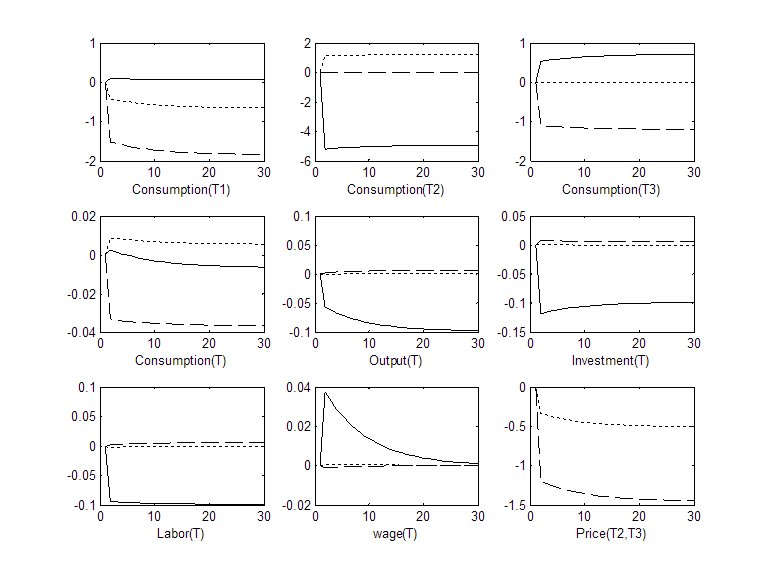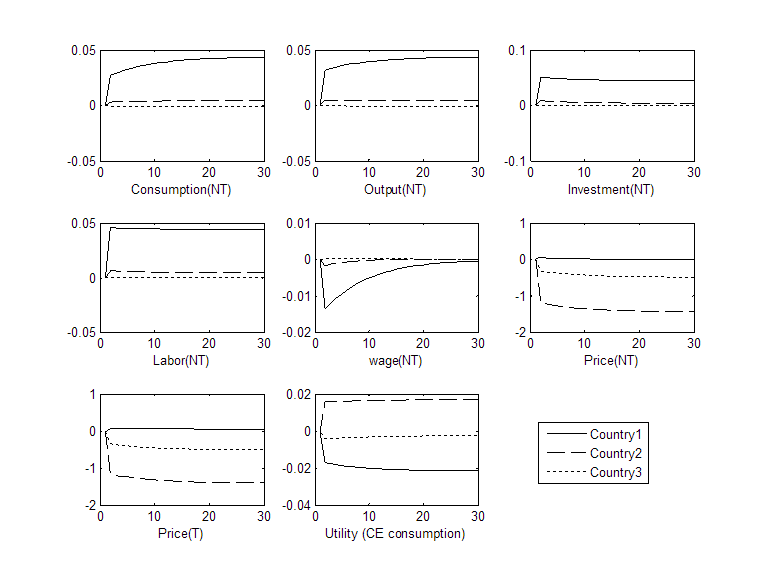Note: horizontal axis displays the number of years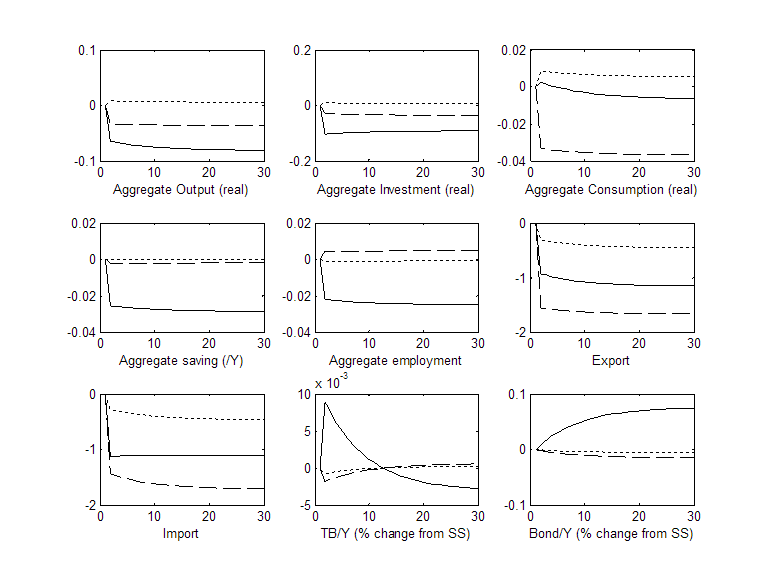Figure 2. Impulse responses of an increase in US tariff rate against all import goods to 10%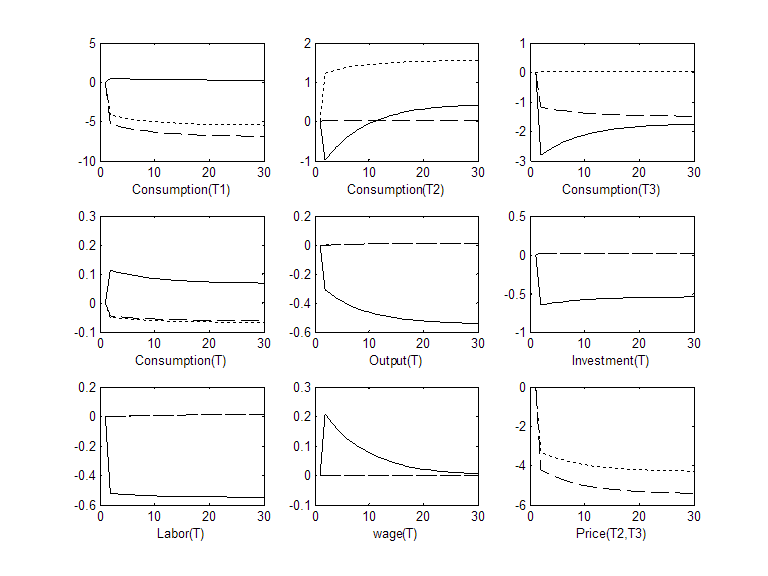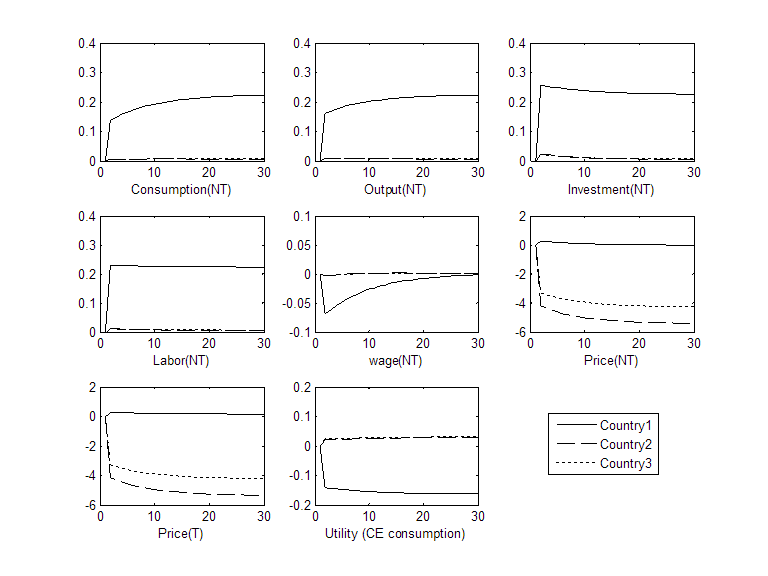Note: horizontal axis displays the number of years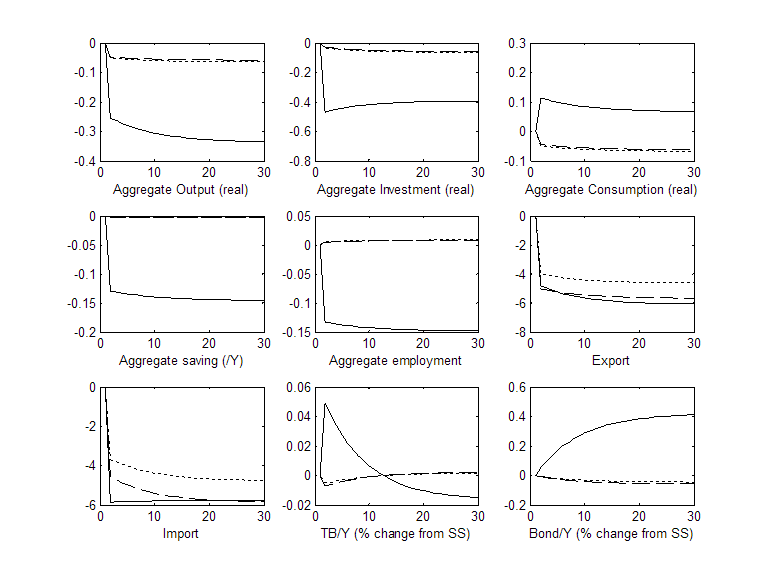Figure 3. Impulse responses of an increase in US tariff rate against Chinese import goods to 10%

When γ=0.5 (high elasticity of substitution in tradable good consumption)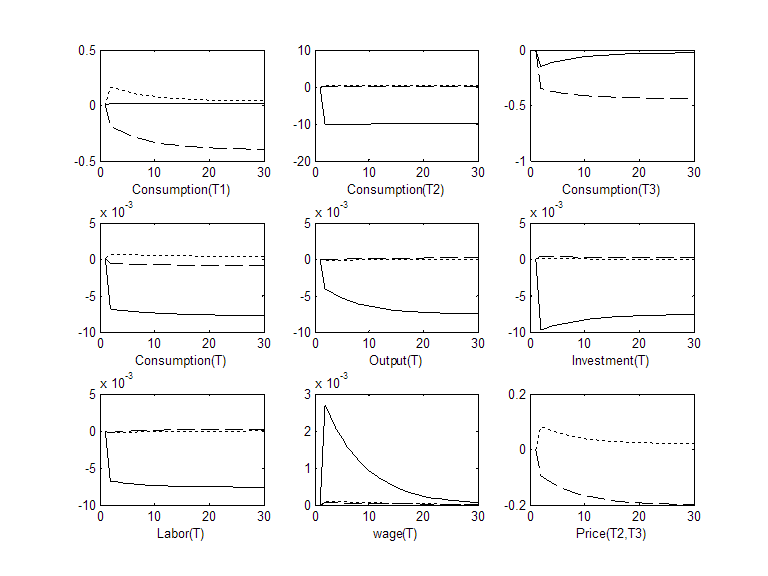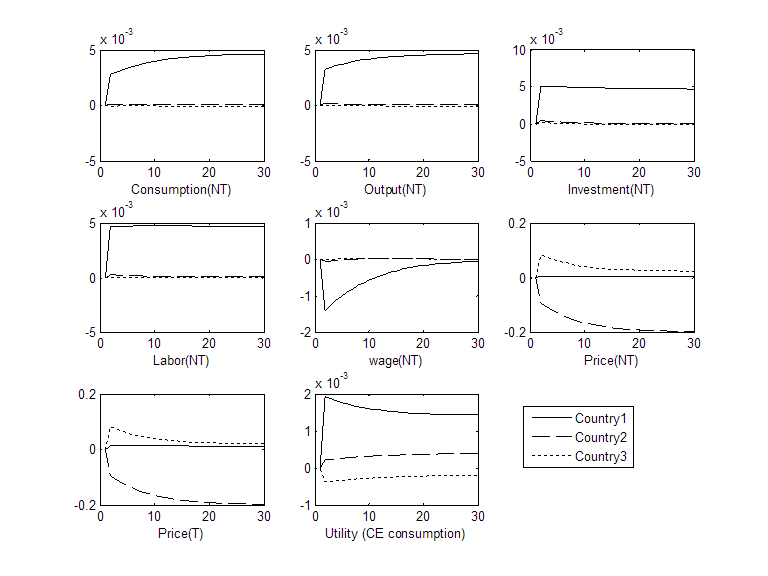Note: horizontal axis displays the number of years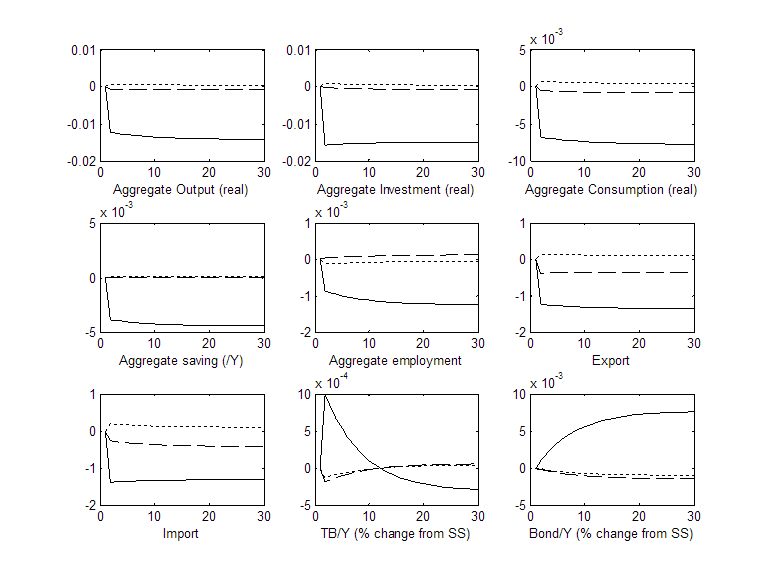Figure 4. Impulse responses of an increase in US tariff rate against all import goods to 10%

When γ=0.5 (high elasticity of substitution in tradable good consumption)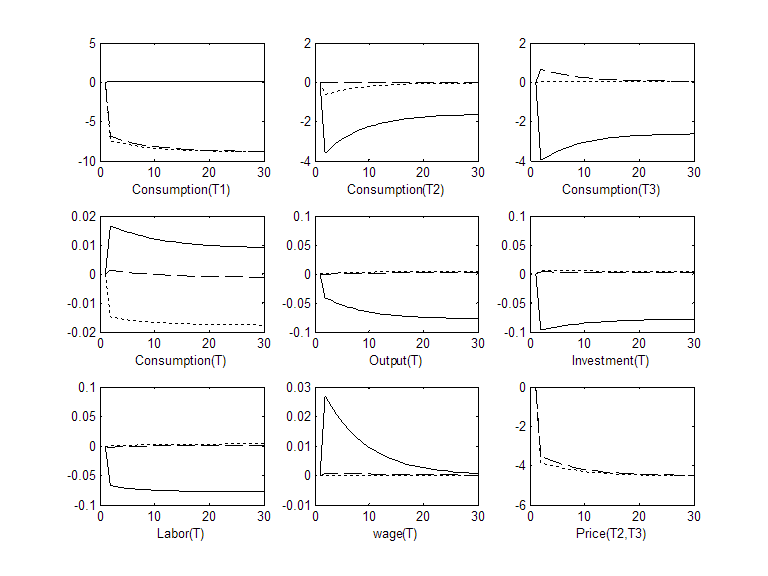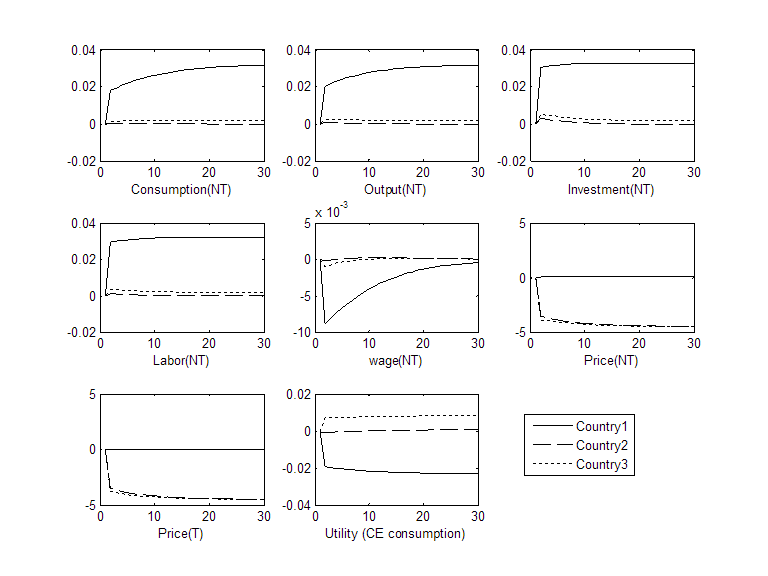Note: horizontal axis displays the number of years